sharps.lv
reklama reklama
 Jūsu IP adrese: 75.101.220.230 Unikālie lietotāji: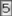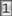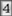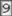Kopējie apmeklējumi: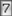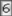Tavi apmeklējumi: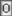Apmeklētās lapas: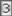===Mani Favoriti=== sharps.lv
SHARPS.LV

====Komponentes====

Kondensatori

sharps.lv

Kondensatoru kodēšana:

There is a three digit code printed on a ceramic capacitor specifying its value. The first two digits are the two significant figures and the third digit is a base 10 multiplier. The value is given in picofarads (pF). A letter suffix indicates the tolerance

C M 0.25pF 20% 0.5pF +100 -0% 5% -20 +50% 10% -20 + 80%

Example: a label of "104K" indicates 10104 pF = 100,000 pF = 100 nF = 0.1uF (aka 0.mF) 10%

There is also an EIA three character code that indicates temperature coefficient. For non-temperature-compensating capacitor, the code consists of three letters. The first character is a letter that gives the low-end operating temperature. The second is a digit gives the high-end operating temperature. The final letter gives capacitance change over that temperature range:

Letter (low temp) Digit (high temp) Letter (change)
X= -55C (-67F) 2= +45C (+113F) D= 3.3%
Y= -30C (-22F) 4= +65C (+149F) E= 4.7%
Z= +10C (+50F) 5= +85C (+185F) F= 7.5%
- 6=+105C (+221F) P= 10%
- 7=+125C (+257F) R= 15%
- - S= 22%
- - T= +22 to -33%
- - U= +22 to -56%
- - V= +22 to -82%

For instance, a Z5U capacitor will operate from +10C to +85C with a capacitance change of at most +22% to -56%. An X7R capacitor will operate from -55C to +125C with a capacitance change of at most 15%.

Temperature-compensated capacitors use a different EIA code. Here, the first letter gives the significant figure of the change in capacitance over temperature in ppm/C. The second character gives the multiplier. The third character gives the maximum error from that in PPM/C. All ratings are from 25-85C:

Significant Figure Multiplier Tolerance
C: 0.0 0: -1 G: 30
B: 0.3 1: -10 H: 60
L: 0.8 2: -100 J: 120
A: 0.9 3: -1000 K: 250
M: 1.0 4: +1 L: 500
P: 1.5 6: +10 M: 1000
R: 2.2 7: +100 N: 2500
S: 3.3 8: +1000 -
T: 4.7 - -
V: 5.6 - -
U: 7.5 - -

For instance, a C0G will have 0 drift, with an error of 30PPM/C, while a P3K will have -1500PPM/C drift, with a maximum error of 250PPM/C.

Note that in addition to the EIA capacitor codes, there are industry capacitor codes and military capacitor codes.